Courses

# Test: Footprints - 2

## 20 Questions MCQ Test Mathematics (Maths) for Class 2 | Test: Footprints - 2

Description
This mock test of Test: Footprints - 2 for Class 2 helps you for every Class 2 entrance exam. This contains 20 Multiple Choice Questions for Class 2 Test: Footprints - 2 (mcq) to study with solutions a complete question bank. The solved questions answers in this Test: Footprints - 2 quiz give you a good mix of easy questions and tough questions. Class 2 students definitely take this Test: Footprints - 2 exercise for a better result in the exam. You can find other Test: Footprints - 2 extra questions, long questions & short questions for Class 2 on EduRev as well by searching above.
QUESTION: 1

### Which two shapes are there in the given figure?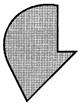Solution: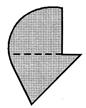The given figure has a quarter circle and a triangle.

QUESTION: 2

### Study the given figure and answer the questions that follow.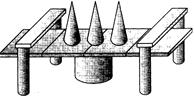Identify the number of cylinders.

Solution:

There are 5 cylinders in the given figure.

QUESTION: 3

### Study the given figure and answer the questions that follow.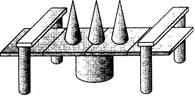How many cones are there?

Solution:

There are 3 cones in the figure.

QUESTION: 4

Study the given figure and answer the questions that follow.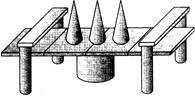How many shapes in all have curved surfaces?

Solution:

3 cones and 5 cylinders i.e., 8 shapes have curved surfaces.

QUESTION: 5

Find the number of triangles in the figure given.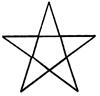Solution:
QUESTION: 6

How many more flat faces does solid A have than Solid B?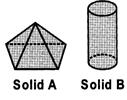Solution:

No. of flat faces in Solid A = 5 No. of flat faces in Solid B = 2
∴ Their difference = 5 ? 2 = 3

QUESTION: 7

Study the given figure and answer the questions that follow.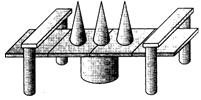How many cuboids are there?

Solution:

There are 12 cuboids in the figure.

QUESTION: 8

Study the given figure and answer the questions that follow.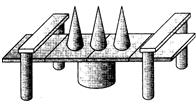How many more cylinders are there than cones?

Solution:

There are 3 cones and 5 cylinders in the figure. So, there are 5 - 3 = 2 more cylinders than cones.

QUESTION: 9

How many squares are there in the. Given figure?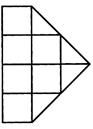Solution:
QUESTION: 10

Find the number of sides in the figure given.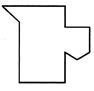Solution:
QUESTION: 11

Which shape is the largest in number in the given figure?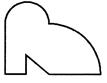Solution:
QUESTION: 12

Identify the shapes in the given figure.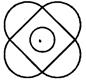Solution: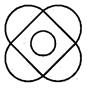The given figure has 4 semicircles, 1 circle and 1 square.

QUESTION: 13

How many more squares than circles are there in the figure?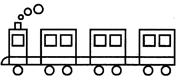Solution:

The no. of circles = 10 The no. of squares = 11
∴ There is 1 square more than the number of circles in the given figure.

QUESTION: 14

Which shape is 2 more than the number of triangles in the picture?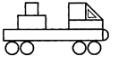Solution:

No. of circles = 4 No. of triangles = 2
∴ There are 2 circles more than the number of triangles.

QUESTION: 15

How many more rectangular faces than square faces does the solid have?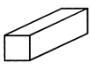Solution:

The number of rectangular faces = 4 The number of square faces = 2
∴ The solid has 4 ? 2 = 2 more square faces than rectangular faces.

QUESTION: 16

What shapes can be seen in the given figure?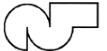Solution:
QUESTION: 17

How many squares are there in the given figure?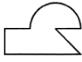Solution: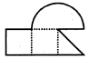QUESTION: 18

Find the correct number of each shape in the given figure.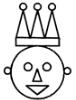Solution: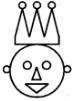The number of circles = 8, the no. of triangles = 5 and the no. of rectangles = 1

QUESTION: 19

How many circles are there in the figure given?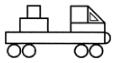Solution:

There are 4 circles in the given figure.

QUESTION: 20

Identify the correct match of the given parts of shapes.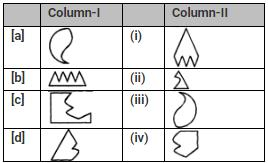Solution:

The correct match of the parts of the given shapes is as in option [a].